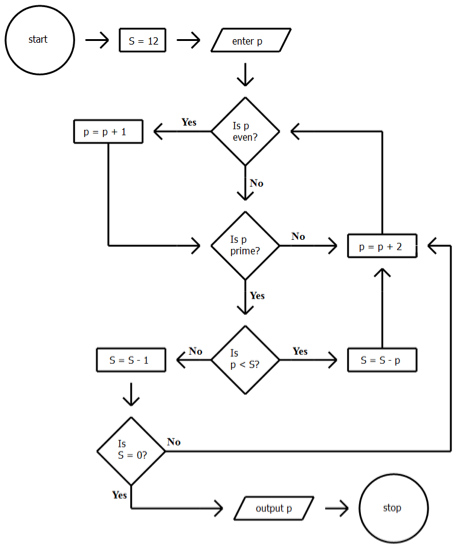Use the dropdown menus to complete each statement according to the information providedThe flowchart represents a mathematical algorithm that takes one positive integer as the input and returns a positive integer as the output. Processes are indicated in the rectangular symbols in the flowchart. Each process is represented by an equation, such as p = p + 1. In this particular process, one is added to the current value of p, and the sum (p + 1) becomes the new value of p. For example, if p = 8 before the process, p = 9 after the process.

A value p = 50 is initially entered. When S first has a value of S = 10, p has a value of

An initial entry that reaches an output in the fewest number of steps is

##### Title

Numerical Flowchart

Correct

Very Hard